paper: Learning Deep Representations By Mutual Information Estimation and Maximization
arxiv：https://arxiv.org/abs/1808.06670
code：https://github.com/rdevon/DIM

# 1 Introduction

• 提出了 Deep InfoMax(DIM)，可以同时估算和最大化输入数据和高级representation之间的互信息(MI)
• 作者提出的最大化互信息的方法，可以根据下游任务是分类还是重建，来对优化全局还是局部的信息进行调整。
• 使用对抗学习约束representation，来使其具有特定于先验的期望统计特征
• 介绍了两种测量 representation 质量的新方法，一个基于Mutual Information Neural Estimation(MINE)$^{}$，一个基于neural dependency measure(NDM)$^{}$，并用它们将DIM与其他无监督学习方法进行比较

# 2 Objectives of Deep InfoMax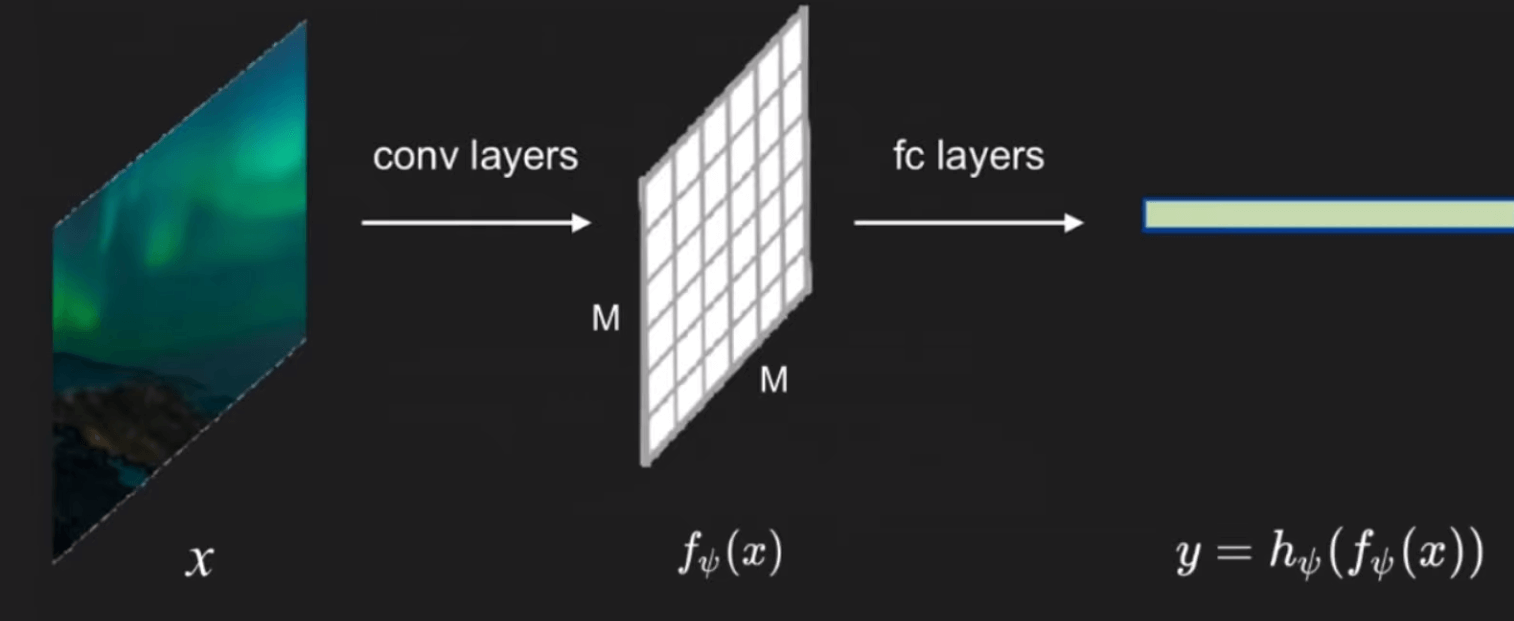$x$ 表示原始图像，$f_{\psi}(x)$ 表示经过卷积层输出的 $M×M$（注意，DIM 里的 $M×M$ 指的是 $M×M$ 块，而不是像素）的 feature map，而 $y=h_{\psi}\left(f_{\psi}(x)\right)$ 则为经过全连接层之后最终的 feature vector（也可以理解为图像的一种 representation），再将这个 representation 应用于一些下游任务，如分类。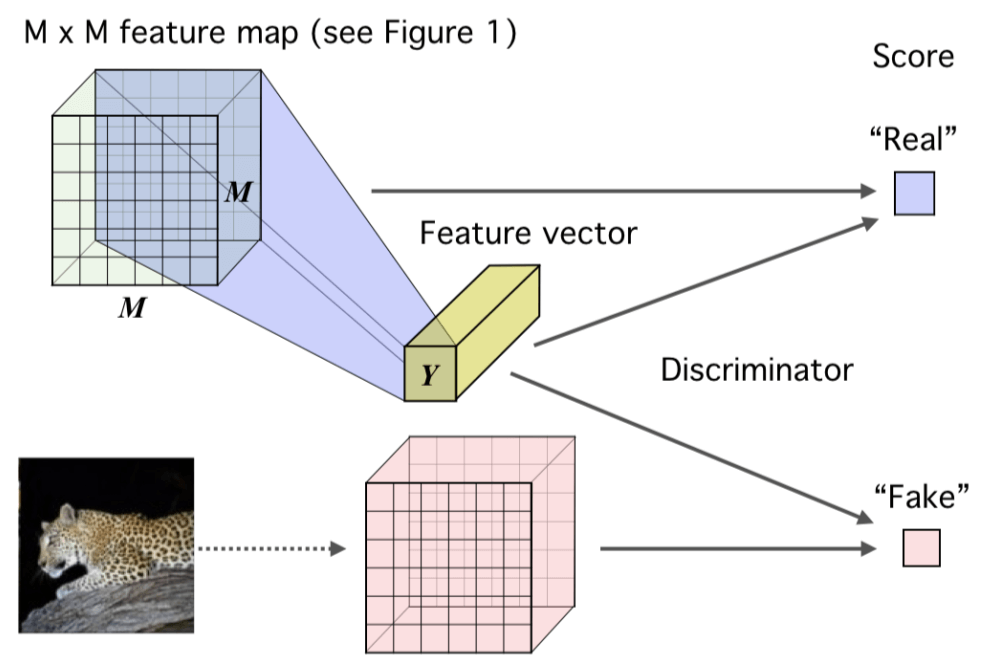- $x∈X$为 image 数据集；编码器 encoder 为 ${ E }_{\psi}=h_{\psi} \circ f_{\psi}$ （ $\circ$ 表示是由后面那两个函数组成 ）； $f_{\psi}(x)$ 表示由将原始图像 $x$ 映射到 feature map； $h_{\psi}(f_{\psi}(x))$ 表示将 feature map 再映射到最后的输出 representation，即 $y=h_{\psi}(f_{\psi}(x))=E_{\psi}(x) ∈ Y$。 - 将样本从 $f_{\psi}(X)$ 经过 $h_{\psi}$ 得到的 $y∈Y$ 的分布，也叫做 ”push-forward distribution“（目前还不是很理解这个名词），记作$Q_{\psi,X}$ 而 DIM 最终的优化目标也就是以下两点： - 最大化 $f_{\psi}(X)$ 和 $Y$ 之间的互信息。（作者根据具体任务的不同，又分为了 global 和 local 两种，后面再提） - 在最终的 representation 中再加入一个统计性的约束，使得到的 $y$ 的分布（push-forward distribution）$Q_{\psi,X}$尽量与先验分布 $Q_{prior}$ 相匹配，有点类似对抗自编码器（AAE）。

# 3 Loss function

### Global Infomax

\begin{aligned} \mathcal I(X;Y) &=\sum_{X, Y} P(X, Y) \log \frac{P(X \mid Y)}{P(X)} \ \end{aligned} \tag 1
KL散度则是衡量同一个随机变量两个分布之间的差异，其值越小，说明分布越接近，定义如下：
\begin{aligned} \mathcal D_{KL}(X||Y) &=\mathbb{E}{P{X Y}}[\log \frac{P(X)}{P(Y)}] \ \end{aligned} \tag 2
MI和KL散度之间的关系也不难推导，如下：

\begin{aligned} \mathcal I(X;Y) &=\sum_{X, Y} P(X, Y) \log \frac{P(X \mid Y)}{P(X)} \\ &=\iint P(X,Y)log \frac{P(X|Y)}{P(X)} \\ &=\mathbb{E}_{P_{X Y}}[log \frac{P(X|Y)}{P(X)}] \\ &=\mathbb{E}_{P_{X Y}}[log \frac{P(X,Y)}{P(X)P(Y)}] \\ &=\mathcal D_{K L}(P_{X Y}||P(X)P(Y)) \end{aligned} \tag 3

$$\mathcal{I}(X ; Y):=\mathcal{D}_{K L}(\mathbb{J}|| \mathbb{M}) \geq \widehat{\mathcal{I}}_{\omega}^{(D V)}(X ; Y):=\mathbb{E}_{\mathbb{J}}\left[T_{\omega}(x, y)\right]-\log \mathbb{E}_{\mathbb{M}}\left[e^{T_{\omega}(x, y)}\right] \tag 4$$ 上式中的 $\mathbb{J}$ 表示 $X$ 和 $Y$ 的联合分布 $P_{X Y}$，$\mathbb{M}$ 表示 $X$ 和 $Y$ 的边缘分布的乘积 $P_{X}P_{y}$，$T_{\omega}$ 表示参数为 $\omega$ 的鉴别器。 更具体来说，这里的 $X$ 为图像的 feature map（ $f_{\psi}(X)$ ），$Y$ 为图像编码后的得到的 representation（$E_{\psi}(X)$）。 $P_{XY}$ 表示图片的 $f_{\psi}(X)$ 和其 $E_{\psi}(X)$ 之间的联合分布（因为来自同一张图片），而 $P_{ \tilde X}P_Y$ 表示另一张图片 $f_{\psi}(\tilde X)$ 和同一个 $E_{\psi}(X)$ 之间这两个边缘分布的乘积（因为来自不同的图片）。**前者需要最大化，而后者需要最小化**。因为鉴别器 $T_{\psi}$ 接收两个参数 $f_{\psi}(X) / f_{\psi}(\tilde X)$ 和 E_{\psi}(X)$，然后输出一个分数，表示有多大的把握确信这个 feature map 是对应于这个 representation的（即来自同一张图片），显然来自同一张图片的输出越大越好，来自不同图片的输出越小越好。 因此最大化$\mathcal I(X;Y)$的目标变成了最大化其的下限$\widehat{\mathcal{I}}_{\omega}^{(D V)}(X ; Y)$，而又转化为了一个对抗训练问题。这样，我们就可以写出这一目标的损失函数了，如下（$\psi$为编码器$E$的参数，$\omega为鉴别器的参数）： \begin{aligned} loss_G&=\underset{\omega, \psi}{\max} \widehat{\mathcal{I}}_{\omega}^{(D V)}(f_{\psi}(X);E_{\psi}(X)) \\ &=\underset{\omega, \psi}{\max} \mathbb{E}_{P_{XY}}\left[T_{\omega}(f_{\psi}(X),E_{\psi}(X))\right]-\log \mathbb{E}_{P_X×P_{\tilde X}}\left[e^{T_{\omega}(f_{\psi}(X),E_{\psi}(X))}\right] \\ &=\underset{\omega, \psi}{\min} -(\mathbb{E}_{P_{XY}}\left[T_{\omega}(f_{\psi}(X),E_{\psi}(X))\right]-\log \mathbb{E}_{P_X×P_{\tilde X}}\left[e^{T_{\omega}(f_{\psi}(X),E_{\psi}(X))}\right]) \end{aligned} \tag 5 但由于我们的优化目标并不需要知道 MI 的具体估计值，而只需要能够将其最大化即可，所以我们不一定需要使用 KL 散度（也就是基于 DV representation 的方法），因此将上述损失函数可以写成更广泛的形式，如下： \begin{aligned} loss_G&=\underset{\omega, \psi}{\max}\widehat{\mathcal{I}}_{\omega}\left(f_{\psi}(X) ; E_{\psi}({X})\right) \\ &=\underset{\omega, \psi}{\min}-\widehat{\mathcal{I}}_{\omega}\left(f_{\psi}(X) ; E_{\psi}({X})\right) \end{aligned} \tag 6 式子中的\widehat{\mathcal{I}}_{\omega}$可以换成其他的 MI 估计器，只要能给出 MI 的边界将其进行最大化即可。在文章中，作者尝试了 Jensen-Shannon MI estimator$^{}$和 infoNCE$^{}$两种方法，如下，并对其各自的优势与性能进行了比较分析： $$\widehat{\mathcal{I}}_{\omega, \psi}^{(\mathrm{JSD})}\left(X ; E_{\psi}(X)\right):=\mathbb{E}_{\mathbb{P}}\left[-\operatorname{sp}\left(-T_{\psi, \omega}\left(x, E_{\psi}(x)\right)\right)\right]-\mathbb{E}_{\mathbb{P} \times \tilde{\mathbb{P}}}\left[\operatorname{sp}\left(T_{\psi, \omega}\left(x^{\prime}, E_{\psi}(x)\right)\right)\right] \\ ,where \space sp(z)=log(1+e^z) \tag 7$$ $$\widehat{\mathcal{I}}_{\omega, \psi}^{(\mathrm{infoNCE})}\left(X ; E_{\psi}(X)\right):=\mathbb{E}_{\mathbb{P}}\left[T_{\psi, \omega}\left(x, E_{\psi}(x)\right)-\mathbb{E}_{\tilde{\mathbb{P}}}\left[\log \sum_{x^{\prime}} e^{T_{\psi, \omega}\left(x^{\prime}, E_{\psi}(x)\right)}\right]\right] \tag 8$$ 可以看到，我们上面得到的损失函数有一个下标$G$，实际上就是代表这是 Global 情况下的目标。所谓 Gloabl ，也就是说我们是直接最大化整个图像的输入和输出之间的互信息的，在说什么是 Local Infomax 之前，再总结一下 Global Infomax 的流程： （1）从数据集中取样原始图像$x_+^{(1)},\dots,x_+^{(n)} \sim P_X$，然后计算 feature map$f_{\psi}(x_+^{(i)}) \forall_i $（2）计算图像的 representation$y^{(i)}=h_{\psi}(f_{\psi}(x_+^{(i)}))$（3）将${(f_{\psi}(x_+^{(i)}),y^{i})}$组成正样本对 （4）从数据集中取样不同的图像$x_{-}^{(1)},\dots,x_{-}^{(n)} \sim P_X$，然后计算 feature map$f_{\psi}(x_{-}^{(i)}) \forall_i $（5）将${(f_{\psi}(x_-^{(i)}),y^{i})}$组成负样本对 （6）通过优化编码器的参数$\psi$和鉴别器的参数$\omega$来最小化$-(\frac{1}{n} \sum_{i=1}^{n}T_{\psi}(f_{\psi}(x_+^{(i)}),y^{(i)}))-log \frac{1}{n} \sum_{i=1}^{n} e^{T_{\psi}(f_{\psi}(x_-^{(i)}),y^{(i)}))})$### Local Infomax 先说一下为什么要提出 Local Infomax？直觉上来看，对于一张图片来说，如果我们的下游任务不是重建类的任务，只是对图片进行分类，那么就没有必要对一些琐碎或者对分类任务无关紧要的像素。而如果我们的目标是最大化整张输入图片的 feature map 与 representation，那么编码器为了符合最后的全局最优情况，就有可能会选择到这些对下游任务并无实际作用的部分进行编码，这样得到的 representation 就肯定不会是针对下游任务最优的 representation。 而 Local Infoamx 的思想就是，我们并不将整张图片的 feature map 一次性输入损失函数来进行 MI 最大化，而是将其分为$M×M$块（$M$不是指像素，而是指被分成了$M^2$个块），一次输入一个块和同一个 representation，最终目标是使这$M^2$个块和整张图片的 representation 的平均 MI 达到最大。这样就可以使给每个块之间共享的一些信息进行编码。 文章中也用实验证明了，根据下游任务的不同，Local Inofmax 在图像分类等一些下游任务中确实具有更好的效果。 因此，也就很容易写出 Local Infomax 的损失函数（与$loss_G$的$\omega不是同一个）： \begin{aligned} loss_L&=\underset{\omega, \psi}{\max} \frac{1}{M^{2}} \sum_{i=1}^{M^{2}} \widehat{\mathcal{I}}_{\omega, \psi}\left(f_{\psi}^{(i)}(X) ; E_{\psi}(X)\right) \\ &=\underset{\omega, \psi}{\min} \frac{1}{M^{2}} -\sum_{i=1}^{M^{2}} \widehat{\mathcal{I}}_{\omega, \psi}\left(f_{\psi}^{(i)}(X) ; E_{\psi}(X)\right) \end{aligned} \tag 9 总结一下 Local Infomax 的流程： （1）从数据集中取样原始图像x_+^{(1)},\dots,x_+^{(n)} \sim P_X$，然后计算 feature map$f_{\psi}(x_+^{(i)}) \space \forall_i $（2）计算图像的 representation$y^{(i)}=h_{\psi}(f_{\psi}(x_+^{(i)})) \space \forall_i$（3）将${(f_{\psi}(x_+^{(i)})_j,y^{i})} \space \forall_j$组成正样本对 （4）从数据集中取样不同的图像$x_{-}^{(1)},\dots,x_{-}^{(n)} \sim P_X$，然后计算 feature map$f_{\psi}(x_{-}^{(i)}) \forall_i $（5）将${(f_{\psi}(x_-^{(i)})_j,y^{i})} \space \forall_j$组成负样本对 （6）通过优化编码器的参数$\psi$和鉴别器的参数$\omega$来最小化$-\frac{1}{M^2} \sum_{j=1}^{M^2}(\frac{1}{n} \sum_{i=1}^{n}T_{\psi}(f_{\psi}(x_+^{(i)})_j,y^{(i)}))-log \frac{1}{n} \sum_{i=1}^{n} e^{T_{\psi}(f_{\psi}(x_-^{(i)})_j,y^{(i)}))})$看起来和 Global Infomax 的流程差不多，主要是在第（3）（5）步中，现在需要对每个 patch 执行此操作（也就是Global的$M^2$倍） ，而之所以只用$j$来对 patch 进行索引，是因为 patch 在哪一行哪一列并不重要。第（5）步中的每个patch$j$则是来自另一张不同的图像。 Local Infomax的原理图如下：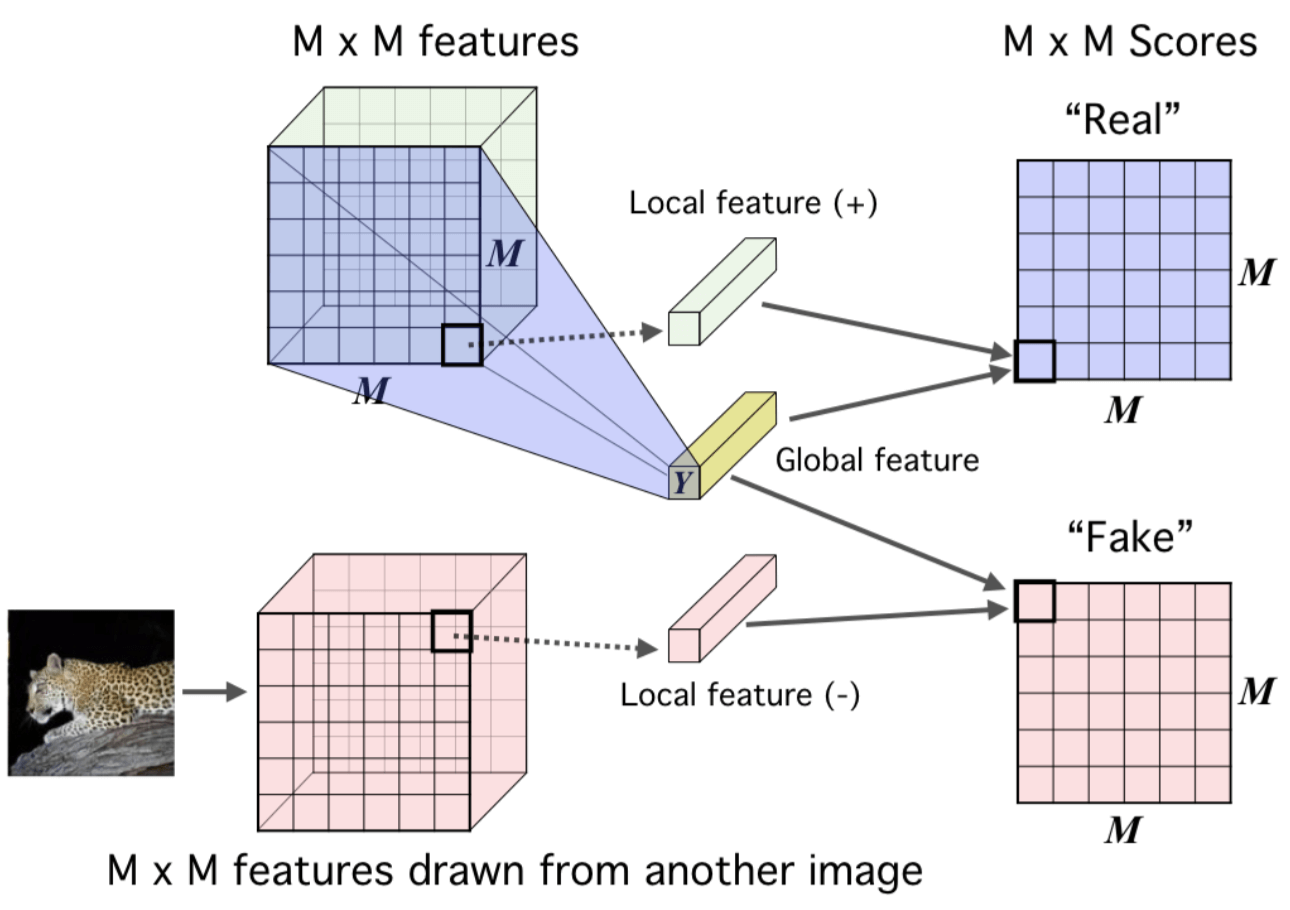至于怎么计算这个平均互信息，文章的附录中给了两种方法，一个是直接将最后的 representation（$Y$）复制然后接到 feature map(+) 的块后面形成real，接到 feature map(-) 后面形成 fake，然后再利用一个$1×1$的卷积鉴别器对对进行评分，如下图：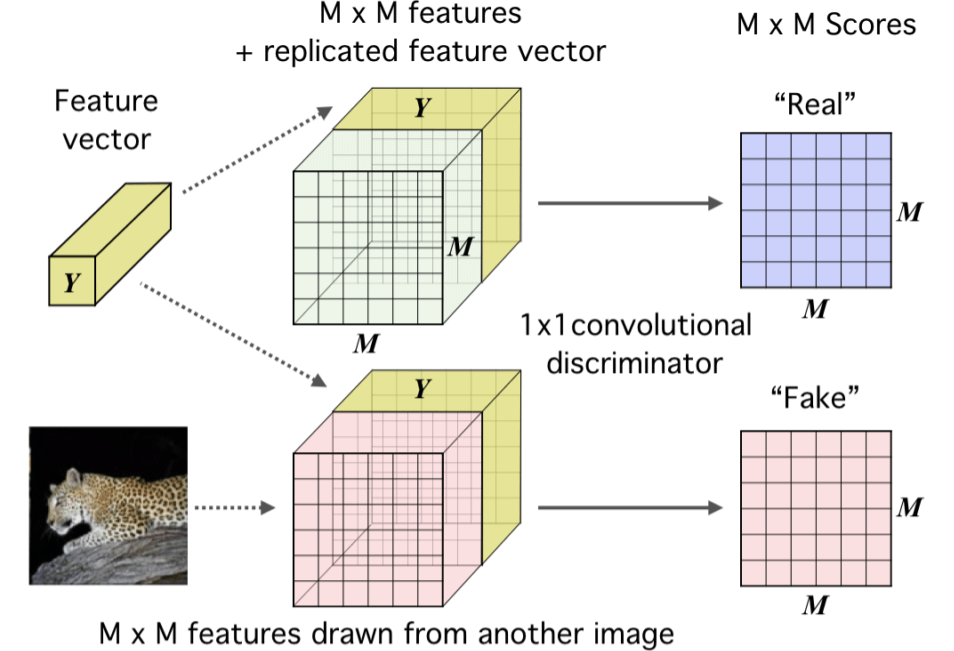另一种方法则是利用点击运算，即对 representation（$Y$）进行全连接网络的编码，每个块用一个$1×1$的网络进行编码，最后得到的两个结果是想同纬度的，然后进行点乘操作得到对应块的分数：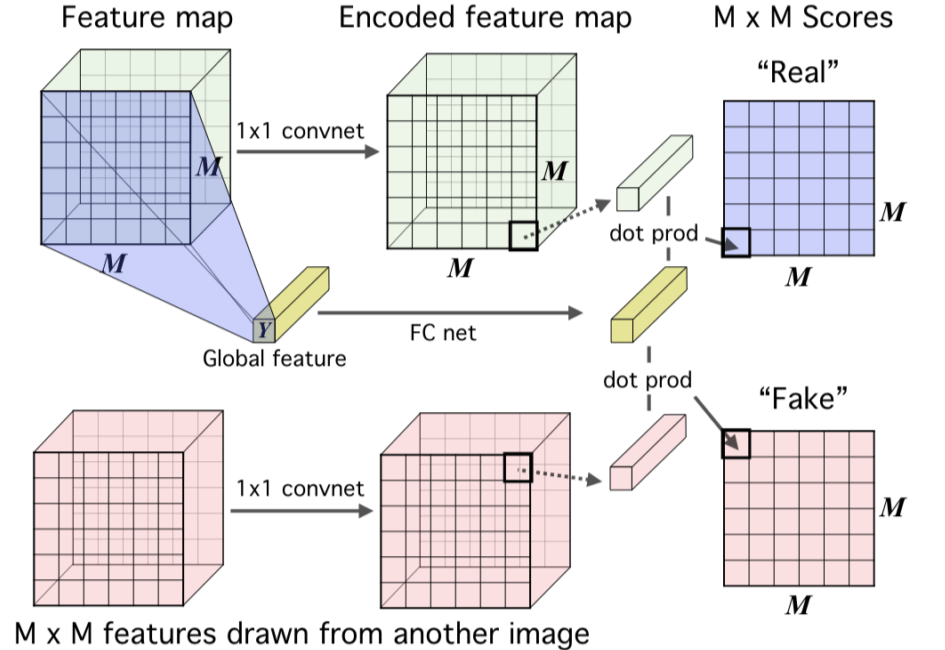### Matching representations to prior 若学习到的隐变量服从标准正态分布的先验分布，这有利于使得编码空间更加规整，甚至有利于解耦特征，便于后续学习。因此，在 DIM 中，我们同样希望加上这个约束，作者利用对抗自编码器（AAE）的思路引入对抗来加入这个约束，即训练一个新的鉴别器，而将编码器当做生成器。鉴别器的目标是区分 representation 分布的真伪（即是否符合先验分布），而编码器则是尽量欺骗判别器，输出更符合先验分布的 representation，如下图：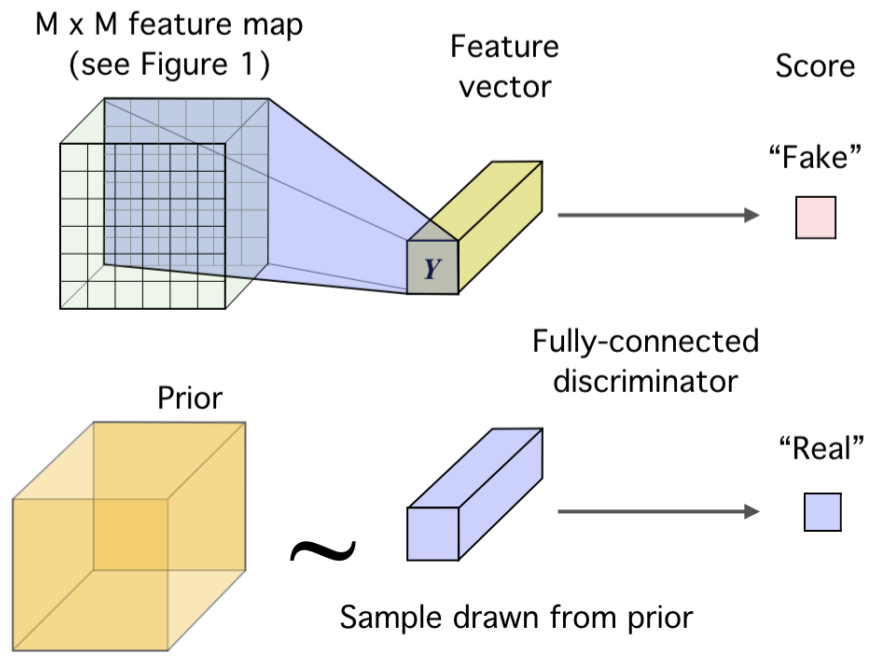具体做法是，训练另一个鉴别器$D_{\phi}$，我们需要学习到一种 representation 来让这个鉴别器$D_{\phi}$确信其来自先验分布$Q_{prior}，这不就是一种对抗的思想。 训练该鉴别器的损失函数如下： \begin{aligned} loss_{matching}&=\underset{\psi}{ \min } \underset{\phi}{ \max } \widehat{\mathcal{D}}_{\phi}\left(Q_{prior} \| Q_{\psi,X}\right) \\ &=\underset{\psi}{ \min } \underset{\phi}{ \max } \mathbb{E}_{Q_{prior}}\left[\log D_{\phi}(y)\right]+\mathbb{E}_{Q_{\psi,X}}\left[\log \left(1-D_{\phi}\left(E_{\psi}(x)\right)\right)\right] \end{aligned} \tag {10} ### Final loss 将上面三个目标整合后，也就得到了 DIM 的最终目标，如下： \begin{aligned} &\underset{\omega_1, \psi}{\max} \alpha \widehat{\mathcal{I}}_{\omega_1}(f_{\psi}(X);E_{\psi}(X)) \space\space\space\space\space\space\space\space\space\space\space\space\space\space\space\space\space\space\space\space\space\space\space\space (globa \space InfoMax)\\ &+\underset{\omega_2, \psi}{\max} \frac{\beta}{M^{2}} \sum_{i=1}^{M^{2}} \widehat{\mathcal{I}}_{\omega_2, \psi}\left(f_{\psi}^{(i)}(X) ; E_{\psi}(X)\right) \space\space\space (local \space InfoMax)\\ &+\underset{\psi}{ \min } \underset{\phi}{ \max } {\gamma}\widehat{\mathcal{D}}_{\phi}\left(Q_{prior} \| Q_{\psi,X}\right) \space\space\space\space\space\space\space\space\space\space\space\space\space\space\space\space\space\space (prior \space matching) \\ &=\underset{\omega_1,\omega_2,\psi}{\min} (-\alpha\widehat{\mathcal{I}}_{\omega_1}(f_{\psi}(X);E_{\psi}(X)) - \frac{\beta}{M^{2}}\sum_{i=1}^{M^{2}} \widehat{\mathcal{I}}_{\omega_2, \psi}\left(f_{\psi}^{(i)}(X) ; E_{\psi}(X)\right)) + \underset{\psi}{ \min } \underset{\phi}{ \max } {\gamma}\widehat{\mathcal{D}}_{\phi}\left(Q_{prior} \| Q_{\psi,X}\right) \end{aligned} 之所以加上\alpha、\beta、\gamma$三个参数，是因为有时候我们只想使用 global InfoMax (如重建类下游任务)，就可以将$\beta$设置为0；而有时候只想使用 Iocal InfoMax (如分类任务)，就可以将$\alpha$设置为0；但这两种情况下，最佳的$\gamma$是不同的，所以也需要$\gamma\$ 来进行调节。

 Ishmael Belghazi, Aristide Baratin, Sai Rajeswar, Sherjil Ozair, Y oshua Bengio, Aaron Courville, and R Devon Hjelm. Mine: mutual information neural estimation. arXiv preprint arXiv:1801.04062, ICML’2018, 2018.

 Alireza Makhzani, Jonathon Shlens, Navdeep Jaitly, Ian Goodfellow, and Brendan Frey. Adversarial autoencoders. arXiv preprint arXiv:1511.05644, 2015.

 Anthony J Bell and Terrence J Sejnowski. An information-maximization approach to blind separation and blind deconvolution. Neural computation, 7(6):1129–1159, 1995.

 Philemon Brakel and Y oshua Bengio. Learning independent features with adversarial nets for non-linear ica. arXiv preprint arXiv:1710.05050, 2017.

 Sebastian Nowozin, Botond Cseke, and Ryota Tomioka. f-gan: Training generative neural samplers using variational divergence minimization. In Advances in Neural Information Processing Systems, pp. 271–279, 2016.

 Aaron van den Oord, Yazhe Li, and Oriol Vinyals. Representation learning with contrastive predictive coding. arXiv preprint arXiv:1807.03748, 2018.